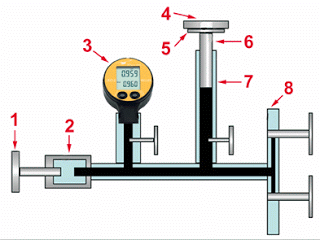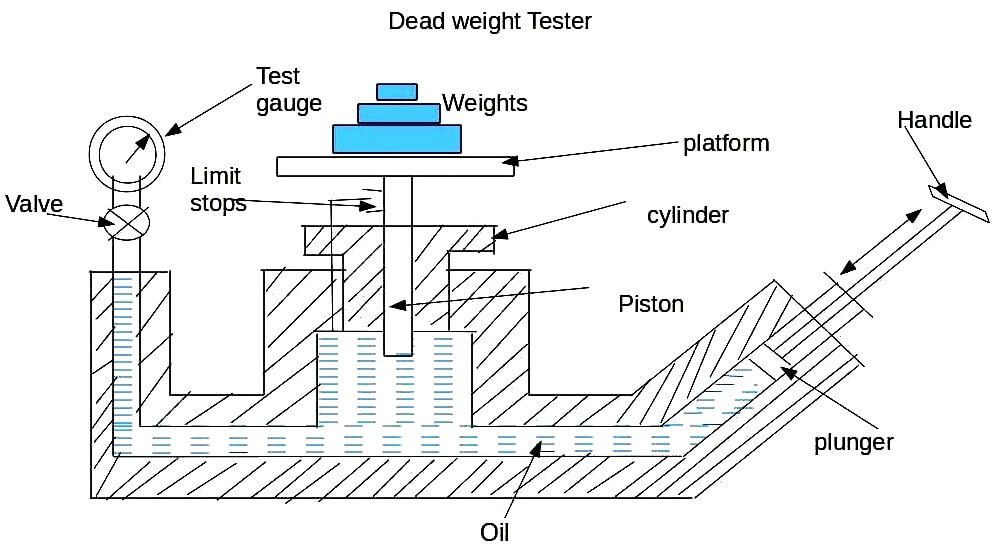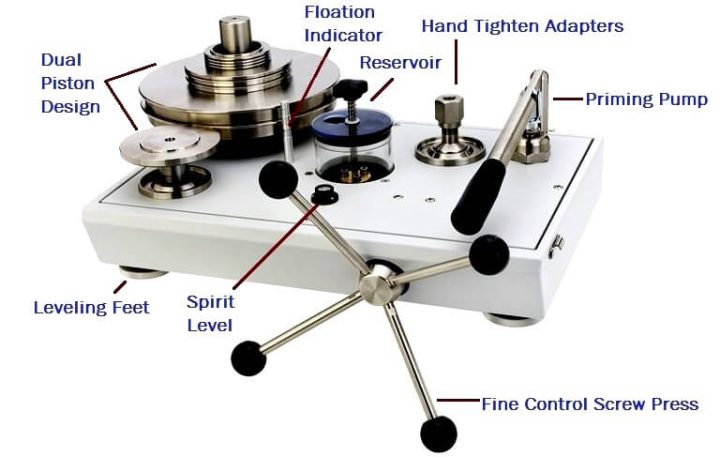# What is a Dead Weight Tester?

A dead weight tester is an instrument that calibrates pressure by determining the weight of force divided by the area the force is applied.

The formula for dead weight testers is pressure equals force divided by area of where force is applied.Dead weights are usually used for pressure gauge calibration as they come with high accuracy, So they can be used as primary standard (as mentioned before).there are many types of them depending on the application and they are operated with oil (hydraulic) or with air (pneumatic).

Dead weight testers are the basic primary standard for accurate measurement of pressure.

Dead weight testers are used to measure the pressure exerted by gas or liquid and can also generate a test pressure for the calibration of numerous pressure instruments.

In dead weight tester, we put the weight on the weight stand of dead weight tester putting weight is reference weight which is to be calibrate and further we applied pressure by moving piston ,when applied pressure and reference weight(Pressure)is equal at this condition reference weight will be zero(Dead). therefore it is called dead weigh tester.

A deadweight tester (DWT) is a calibration standard which uses a piston cylinder on which a load is placed to make an equilibrium with an applied pressure underneath the piston.

The formula to design a DWT is based basically is expressed as follows :

p = F / A     [Pa]

where :

p : reference pressure [Pa]
F : force applied on piston [N]
A : effective area PCU [m2]

### Working of Dead Weight Tester :1 – Hand pump

2 – Testing Pump

3 – Pressure Gauge to be calibrated

4 – Calibration Weight

5 – Weight Support

6 – Piston

7 – Cylinder

8 – Filling Connection

### Basics:

Dead weight testers are a piston-cylinder type measuring device. As primary standards, they are the most accurate instruments for the calibration of electronic or mechanical pressure measuring instruments.

They work in accordance with the basic principle that P= F/A, where the pressure (P) acts on a known area of a sealed piston (A), generating a force (F).

The force of this piston is then compared with the force applied by calibrated weights. The use of high quality materials result in small uncertainties of measurement and excellent long term stability.

Dead weight testers can measure pressures of up to 10,000 bar, attaining accuracies of between 0.005% and 0.1% although most applications lie within 1 – 2500 bar.

The pistons are partly made of tungsten carbide (used for its small temperature coefficient), and the cylinders must fit together with a clearance of no more than a couple of micrometers in order to create a minimum friction thus limiting the measuring error. The piston is then rotated during measurements to further minimize friction.

The testing pump (2) is connected to the instrument to be tested (3), to the actual measuring component and to the filling socket.

A special hydraulic oil or gas such as compressed air or nitrogen is used as the pressure transfer medium. The measuring piston is then loaded with calibrated weights (4). The pressure is applied via an integrated pump (1) or, if an external pressure supply is available, via control valves in order to generate a pressure until the loaded measuring piston (6) rises and ‘floats’ on the fluid. This is the point where there is a balance between pressure and the mass load.

The piston is rotated to reduce friction as far as possible. Since the piston is spinning, it exerts a pressure that can be calculated by application of a derivative of the formula P = F/A.

The accuracy of a pressure balance is characterized by the deviation span, which is the sum of the systematic error and the uncertainties of measurement.

Today’s dead weight testers are highly accurate and complex and can make sophisticated physical compensations.

They can also come accompanied by an intelligent calibrator unit which can register all critical ambient parameters and automatically correct them in real time making readings even more accurate.

### Operation Procedure

The dead weight tester apparatus consists of a chamber which is filled with oil free impurities and a piston – cylinder combination is fitted above the chamber as shown in diagram. The top portion of the piston is attached with a platform to carry weights.

A plunger with a handle has been provided to vary the pressure of oil in the chamber. The pressure gauge to be tested is fitted at an appropriate plate.

the dead weight tester is basically a pressure producing and pressure measuring device. It is used to calibrate pressure gauges.

The following procedure is adopted for calibrating pressure gauges. Calibration of pressure gauge means introducing an accurately known sample of pressure to the gauge under test and then observing the response of the gauge.

In order to create this accurately known pressure, the following steps are followed. The valve of the apparatus is closed.A known weight is placed on the platform. Now by operating the plunger, fluid pressure is applied to the other side of the piston until enough force is developed to lift the piston-weight combination.

When this happens, the piston weight combination floats freely within the cylinder between limit stops. In this condition of equilibrium, the pressure force of fluid is balanced against the gravitational force of the weights puls the friction drag.

Therefore, PA = Mg + F

Hence : P = Mg + F / A

where, P = pressure

M = Mass; Kg

g = Acceleratoion due to gravity ; m/s²

F = Friction drag; N

A = Eqivalent area of piston – cylinder combination; m²

Thus the pressure P which is caused due to the weights placed on the platform is calculated. After calculating P , the plunger is released.

Now the pressure gauge to be calibrated is fitted at an appropriate place on the dead weight tester. The same known weight which was used to calucated P is placed on the platform.

Due to the weight, the piston moves downwards and exerts a pressure P on the fluid. Now the valve in the apparatus is opened so that the fluid pressure P is transmitted to the gauge, which makes the gauge indicate a pressure value.

This pressure value shown by the gauge should be equal to the known input pressure P. If the gauge indicates some other value other than p the gauge is adjusted so that it reads a value equal to p. Thus the gauge is calibrated.Applications:

It is used to calibrated all kinds of pressure gauges such as industrial pressure gauges, pressure transmitters etc.

it is simple in construction and easy to use. It can be used to calibrated a wide range of pressure measuring devices.

Fluid pressure can be easily varied by adding weights or by changing the piston cylinder combination.

Limitations:

the accuracy of the dead weight tester is affected due to the friction between the piston and cylinder, and due to the uncertainty of the value of gravitational constant ‘g’.

Be the first to get exclusive content straight to your email.
We promise not to spam you. You can unsubscribe at any time.

### 6 thoughts on “What is a Dead Weight Tester?”

1.Before I am searching other websites (matter not clear), But your website mater is very deap and clear.
Thank you once again……..

•deep…..

2.3.Excellent narration.

4.5.